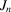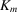International
Tables for
Crystallography
Volume B
Reciprocal space
Edited by U. Shmueli

International Tables for Crystallography (2006). Vol. B. ch. 2.1, pp. 198-199   | 1 | 2 |

## Section 2.1.6.4. The use of normal approximations

U. Shmuelia* and A. J. C. Wilsonb

aSchool of Chemistry, Tel Aviv University, Tel Aviv 69 978, Israel, and bSt John's College, Cambridge, England
Correspondence e-mail:  ushmueli@post.tau.ac.il

#### 2.1.6.4. The use of normal approximations

| top | pdf |

Sinceand[equations (2.1.6.1)and (2.1.6.8)] are sums of identically distributed variables conforming to the conditions of the central-limit theorem, it is tempting to approximate their distributions by normal distributions with the correct mean and variance. This would be reasonably satisfactory for the distributions ofandthemselves for quite small values of n and m, but unsatisfactory for the distribution of their ratio for any values of n and m, even large. The ratio of two variables with normal distributions is notorious for its rather indeterminate mean and `infinite' variance, resulting from the `tail' of the denominator distributions extending through zero to negative values. The leading terms of the ratio distribution are given by Kendall & Stuart (1977, p. 288).

### ReferencesKendall, M. & Stuart, A. (1977). The advanced theory of statistics, Vol. 1, 4th ed. London: Griffin.Google Scholar